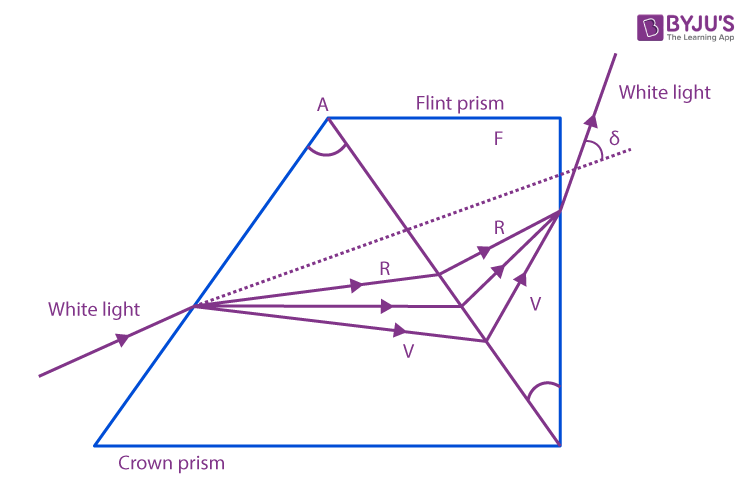# Dispersion Without Deviation

Dispersion without deviation is one of the most important concepts in optics. We know light is a form of energy that can undergo various phenomena. Dispersion and deviation are some of the phenomena of light. The condition of dispersion without deviation is achieved using flint glass prisms. Let us have a look at what is meant by dispersion, deviation, and how dispersion without deviation is achieved.

### Dispersion and Deviation

Dispersion is the concept seen when white light is passed through a prism. The light splits into its spectrum of colours, in the order of violet, indigo, blue, green, yellow, orange and red (VIBGYOR). This process in which white light splits into its constituent colours is known as dispersion. Example: Dispersion is seen in the formation of a rainbow.

Deviation can be observed when light travels from one medium to another medium. The light deviates from its original path in the previous medium due to a change in its velocity when traveling through the new medium. This is known as the refraction of light.

Now, let us know more about dispersion without deviation.

## Dispersion Without Deviation Derivation

When white light is incident on a crown glass prism, it disperses into a band of colours and deviates too. The light deviation produced by the crown glass prism for mean colour is equal and opposite to that produced by the flint glass prism.

To observe dispersion without deviation, two thin prisms are placed in contact with each other as shown in the figure below.We know that deviation produced by a crown glass prism is equal to the deviation produced by a flint glass prism.

Let the refractive indices of crown and flint glass prisms for mean ray be:

Deviation produced for the mean ray by the crown prism is given by:

$$\begin{array}{l}\delta _{\gamma }=(\mu _{\gamma}-1)A\end{array}$$
————– (1)

Deviation produced for the mean ray by the flint prism is given by:

$$\begin{array}{l}\delta^{‘}_{\gamma }=(\mu^{‘}_{\gamma}-1)A^{‘}\end{array}$$
————– (2)

Since resultant deviation is zero, hence

$$\begin{array}{l}\delta_{\gamma}=-\delta^{‘}_{\gamma}\end{array}$$

Substituting values,

$$\begin{array}{l}(\mu _{\gamma}-1)A = – (\mu^{‘} _{\gamma}-1)A^{‘}\end{array}$$

Or

$$\begin{array}{l}A^{‘} = [\frac{(\mu_{\gamma}-1)}{(\mu^{‘}_{\gamma}-1)}]A\end{array}$$
———- (3)

Since the refracting angles of the prisms are in opposite directions to each other, it is indicated by the negative sign.

The resultant dispersions is given by:

The refractive indices of the crown and flint glass prisms for red and violet colour rays are given below.

The angular dispersion for a crown glass prism is given as:

$$\begin{array}{l}\Theta _{1}=(\mu _{v}-\mu _{R})A\end{array}$$
————————– (4)

The angular dispersion fora flint glass prism is given as:

$$\begin{array}{l}\Theta _{2}=(\mu^{‘} _{v}-\mu^{‘} _{R})A^{‘}\end{array}$$
————————– (5)

The resultant angular dispersion is given as

$$\begin{array}{l}\Theta = \Theta _{2}-\Theta _{1}\end{array}$$

Substituting equation (4) in (5)

$$\begin{array}{l}\Theta = (\mu^{‘} _{v}-\mu^{‘} _{R})A^{‘}-(\mu _{v}-\mu _{R})A\end{array}$$

$$\begin{array}{l}\Theta = (\mu^{‘} _{v}-\mu^{‘} _{R})[\frac{(\mu_{\gamma}-1)}{(\mu^{‘}_{\gamma}-1)}]A-(\mu _{v}-\mu _{R})A\end{array}$$

$$\begin{array}{l}\Theta = (\mu_{\gamma}-1)A[\frac{\mu^{‘}_{v} -{\mu}’ _{R}}{{\mu}’ _{\gamma}-1}][\frac{\mu_{v} -{\mu} _{R}}{{\mu} _{\gamma}-1}]\end{array}$$

$$\begin{array}{l}\Theta = (\mu_{\gamma}-1)A (\omega ^{‘}-\omega )\end{array}$$

Where

$$\begin{array}{l}{\omega} = [\frac{\mu_{v} -{\mu} _{R}}{{\mu} _{\gamma}-1}]\end{array}$$

$$\begin{array}{l}{\omega}’ = [\frac{\mu^{‘}_{v} -{\mu}’ _{R}}{{\mu}’ _{\gamma}-1}]\end{array}$$

To know in detail about the prism, watch the video below.Hope you have understood about dispersion without deviation along with derivation. Stay tuned with BYJU’S to know more about various science and math concepts.

## Frequently Asked Questions – FAQs

### What is dispersion?

Dispersion is defined as the separation of white light into different colors when light is passed through a prism.

### What is the deviation of light?

Deviation is the phenomenon that is observed when light travels from one medium to another medium. Deviation of light is due to the phenomenon of refraction.

True.

### What is a prism?

A prism is a three-dimensional solid object in which the two ends are identical. Prism is the combination of the flat faces, identical bases and equal cross-sections.

### Give the formula for dispersion without deviation.

The formula for dispersion without deviation is:

$$\begin{array}{l}\Theta = (\mu_{\gamma}-1)A (\omega ^{‘}-\omega )\end{array}$$